## Selina Concise Mathematics Class 10 ICSE Solutions Chapter 15 Similarity (With Applications to Maps and Models) Ex 15D

These Solutions are part of Selina Concise Mathematics Class 10 ICSE Solutions. Here we have given Selina Concise Mathematics Class 10 ICSE Solutions Chapter 15 Similarity Ex 15D.

Other Exercises

Question 1.
A triangle ABC has been enlarged by scale factor m = 2.5 to the triangle A’ B’ C’. Calculate:
(i) the length of AB, if A’ B’ = 6 cm.
(ii) the length of C’ A’ if CA = 4 cm.
Solution:
Scale factor (k) = 2.5
∆ABC is enlarged to ∆A’B’C’
(i) A’B’ = 6 cm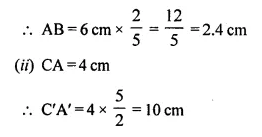Question 2.
A triangle LMN has been reduced by scale factor 0.8 to the triangle L’ M’ N’. Calculate:
(i) the length of M’ N’, if MN = 8 cm.
(ii) the length of LM, if L’ M’ = 5.4 cm.
Solution:
∆LMN has been reduced by the scale factor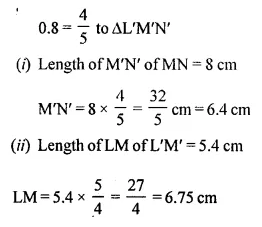Question 3.
A triangle ABC is enlarged, about the point O as centre of enlargement, and the scale factor is 3. Find:
(i) A’ B’, if AB = 4 cm.
(ii) BC, if B’ C’ = 15 cm.
(iii) OA, if OA’= 6 cm.
(iv) OC’, if OC = 21 cm.
Also, state the value of:
(a) $$\frac { OB’ }{ OB }$$
(b) $$\frac { C’A’ }{ CA }$$
Solution:
∆ABC is enlarged to ∆A’B’C’ about the point O as its centre of enlargement.
Scale factor = 3 = $$\frac { 3 }{ 1 }$$Question 4.
A model of an aeroplane is made to a scale of 1 : 400. Calculate:
(i) the length, in cm, of the model; if the length of the aeroplane is 40 m.
(ii) the length, in m, of the aeroplane, if length of its model is 16 cm.
Solution:
Model of an aeroplane to the actual = 1 : 400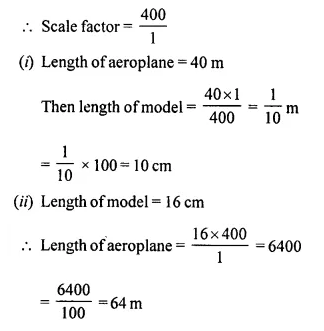Question 5.
The dimensions of the model of a multistorey building are 1.2 m x 75 cm x 2 m. If the scale factor is 1 : 30; find the actual dimensions of the building.
Solution:
Dimensions of a model of multistorey building = 1.2 m x 75 cm x 2 m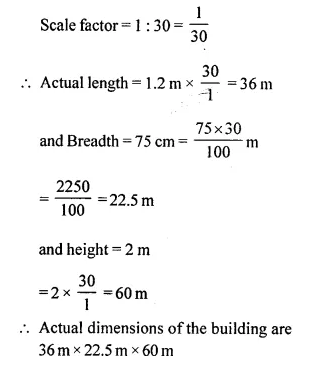Question 6.
On a map drawn to a scale of 1 : 2,50,000; a triangular plot of land has the following measurements : AB = 3 cm, BC = 4 cm and angle ABC = 90°.
Calculate:
(i) the actual lengths of AB and BC in km.
(ii) the area of the plot in sq. km.
Solution:
Scale of map drawn of a triangular plot = 1 : 2,50,000
Measurement of plot AB = 3 cm, BC = 4 cm
and ∠ABC = 90°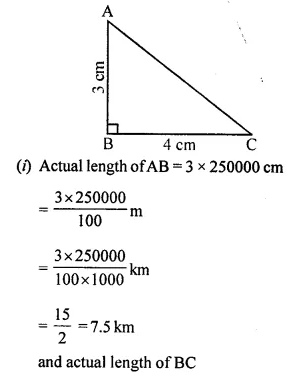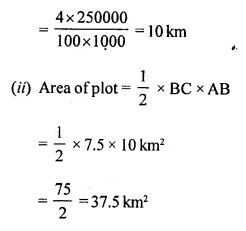Question 7.
A model of a ship of made to a scale 1 : 300
(i) The length of the model of ship is 2 m. Calculate the lengths of the ship.
(ii) The area of the deck ship is 180,000 m². Calculate the area of the deck of the model.
(iii) The volume of the model in 6.5 m3. Calculate the volume of the ship. (2016)
Solution: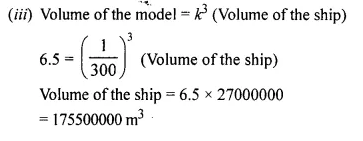Question 8.
An aeroplane is 30 in long and its model is 15 cm long. If the total outer surface area of the model is 150 cm², find the cost of painting the outer surface of the aeroplane at the rate of ₹ 120 per sq.m. Given that 50 sq. m of the surface of the aeroplane is left for windows.
Solution:
Length of aeroplane = 30 m = 3000 cm
and length of its model = 15 cm
Surface area of model = 150 cm²
Scale factor (k) = $$\frac { 3000 }{ 15 }$$ = $$\frac { 200 }{ 1}$$
Area of plane = k² x area of model = (200)² x 150 cm² = 40000 x 150 cm²
$$\frac { 40000 x 150 }{ 10000 }$$ = 600 m² (1 m² = 10000 cm²)
Shape left for windows = 50 sq. m
Balance area = 600 – 50 = 550 sq. m
Race of painting the outer surface = ₹ 120 per sq.m
Total cost = ₹ 550 x 120 = ₹ 66000

Hope given Selina Concise Mathematics Class 10 ICSE Solutions Chapter 15 Similarity Ex 15D are helpful to complete your math homework.

If you have any doubts, please comment below. Learn Insta try to provide online math tutoring for you.